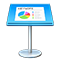# Functions that accept conditions and wildcards as arguments

Some functions, such as SUM, operate on entire ranges. Other functions, such as SUMIF, operate only on the cells in the range that meet a condition. For example, to add up all the numbers in column B that are less than 5, you could use:

=SUMIF(B,“<5”)

The second argument of SUMIF is called a condition because it causes the function to ignore cells that don’t meet the requirements.

There are two types of functions that take conditions:

• Functions that have names ending in IF or IFS (except for the function IF, which doesn’t take a condition; it instead takes an expression that should evaluate to either TRUE or FALSE). These functions can do numeric comparisons in their conditions, such as “>5,” “<=7,” or “<>2.”  These functions also accept wildcards in specifying conditions. For example, to count the number of cells in column B that begin with the letter “a,” you could use:

=COUNTIF(B,“a*”)

• Functions that take conditions, such as HLOOKUP, but can’t do numeric conditions. These functions sometimes permit the use of wildcards.

Functions that allow numeric comparisons or wild cards

Function

Allows numeric comparisons

Accepts wildcards

AVERAGEIF

Yes

Yes

AVERAGEIFS

Yes

Yes

COUNTIF

Yes

Yes

COUNTIFS

Yes

Yes

SUMIF

Yes

Yes

SUMIFS

Yes

Yes

HLOOKUP

No

If exact match specified

MATCH

No

If exact match specified

SEARCH

No

Yes

VLOOKUP

No

If exact match specified